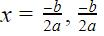Courses

# Test: Equations Reducible to Quadratic Form

## 10 Questions MCQ Test Mathematics (Maths) Class 10 | Test: Equations Reducible to Quadratic Form

Description
This mock test of Test: Equations Reducible to Quadratic Form for Class 10 helps you for every Class 10 entrance exam. This contains 10 Multiple Choice Questions for Class 10 Test: Equations Reducible to Quadratic Form (mcq) to study with solutions a complete question bank. The solved questions answers in this Test: Equations Reducible to Quadratic Form quiz give you a good mix of easy questions and tough questions. Class 10 students definitely take this Test: Equations Reducible to Quadratic Form exercise for a better result in the exam. You can find other Test: Equations Reducible to Quadratic Form extra questions, long questions & short questions for Class 10 on EduRev as well by searching above.
QUESTION: 1

### Ruhi’s mother is 26 years older than her. The product of their ages (in years) 3 years from now will be 360. Form a Quadratic equation so as to find Ruhi’s age​

Solution:

Ruhi’s mother is 26 years older than her
So let Ruhi’s age is x
So mother’s age is x+26
The product of their ages 3 years from now will be 360
So After three years , Ruhi’s age will be x+3
Mother’s age will be x+26+3=x+29
Product of their ages =(x + 3)(x + 29)=360
x2+(3+29)x+87=360
x2+32x-273=0

QUESTION: 2

### Divide 16 into two parts such that twice the square of the larger part exceeds the square of the smaller part by 164.​

Solution:

Let x and (16 - x) are two parts of 16 where (16 - x) is longer and x is smaller .
A/C to question,
2 × square of longer = square of smaller + 164
⇒ 2 × (16 - x)² = x² + 164
⇒ 2 × (256 + x² - 32x ) = x² + 164
⇒ 512 + 2x² - 64x = x² + 164
⇒ x² - 64x + 512 - 164 = 0
⇒ x² - 64x + 348 = 0
⇒x² - 58x - 6x + 348 = 0
⇒ x(x - 58) - 6(x - 58) = 0
⇒(x - 6)(x - 58) = 0
⇒ x = 6 and 58

But x ≠ 58 because x < 16
so, x = 6 and 16 - x = 10

Hence, answer is 6 and 10.

QUESTION: 3

### If ax2 + bx + c, a ≠ 0 is factorizable into product of two linear factors, then roots of ax2 + bx + c = 0 can be found by equating each factor to

Solution:

Roots of the equation means a value of x such that the equation becomes zero when that value is substituted. If the equation can be represented into product of two linear equations then the roots can be found by keeping each of the equation equal to zero.We know two numbers in multiplication is equal to zero only when either of the number is zero.So we get zeros of the equation putting the two linear equations equal to 0

QUESTION: 4

Reduction of a rupee in the price of onion makes the possibility of buying one more kg of onion for Rs.56. Find the original price of the onion per kg?

Solution: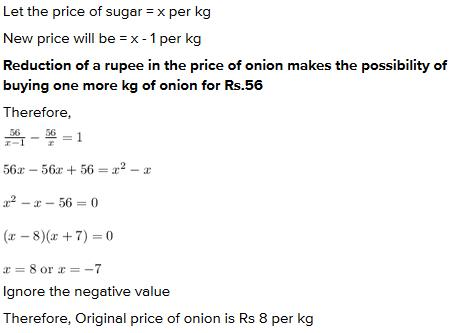QUESTION: 5

What are the two consecutive even integers whose squares have sum 340?​

Solution:
QUESTION: 6

The length of the plot in meters is 1 more than twice its breadth and the area of a rectangle plot is 528m2. Which of the following quadratic equations represents the given situation:​

Solution:
QUESTION: 7

Find the two consecutive odd positive integers, sum of whose square is 290

Solution:
QUESTION: 8

If the length of the rectangle is one more than the twice its width, and the area of the rectangle is 300 square meter. What is the measure of the width of the rectangle?

Solution:
QUESTION: 9

The sum of areas of two squares is 468m2. If the difference of their perimeters is 24m, then the sides of the two squares are:​

Solution:

Let us say that the sides of the two squares are 'a' and 'b'
Sum of their areas = a2 + b2 = 468
Difference of their perimeters = 4a - 4b = 24
=> a - b = 6
=> a = b + 6
So, we get the equation
(b + 6)2 + b2 = 468
=> 2b2 + 12b + 36 = 468
=> b2 + 6b - 216 = 0
=> b = 12
=> a = 18
The sides of the two squares are 12 and 18.

QUESTION: 10

If b2 - 4ac = 0 then The roots of the Quadratic equation ax2 + bx + c = 0 are given by :

Solution:

Formula for finding the roots of a quadratic equation is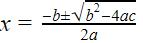So since
b- 4ac = 0, putting this value in the equation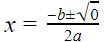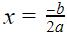So there are repeated roots